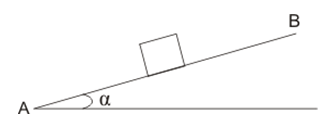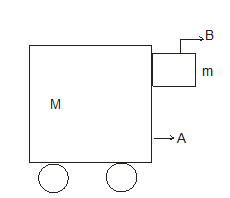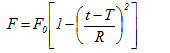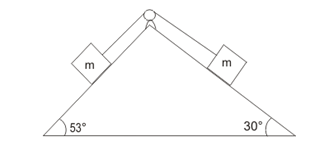# Problems and solution on Force and Newton Law's of Motion for JEE and Other Competitive examinations

In this page we have Problems and solution on Force and Newton Law's of Motion for JEE and Other Competitive examinations . Hope you like them and do not forget to like , social shar and comment at the end of the page.

Question 1
Force exerted on a body changes it's
(a) Direction of motion
(b) Momentum
(c) Kinetic energy
(d) All the above

Question 2
There are two statements
Statement A :Rate of change of momentum corresponds to force
Statement B :Rate of change of momentum corresponds to Kinetic Energy
Which one of the following is correct?
(a) A only
(b) B only
(c) Both A and B are correct
(d) Both A and B are wrong

Question 3
A truck and car are moving on a plane road with same kinetic Energy. They are brought to rest by application of brakes which provide equal retarding forces. Which one of the following statements is true?
(a) Distance traveled by truck is shorter then car before coming to rest
(b) Distance traveled by car is shorter then truck before coming to rest
(c) Distance traveled depends on individual velocity of both the vehicles
(d) Both will travel same distance before coming to rest

Question 4
A block A of mass m1 is released from top of smooth inclined plane and it slides down the plane. Another block of mass m2 such that m2 > m1is dropped from the same point and falls vertically downwards. Which one of the following statements will be true if the friction offered by air is negligible?
(a) Both blocks will reach ground at same time
(b) Both blocks will reach ground with the same speed
(c) speed of both the blocks when they reach ground will depend on their masses
(d) Block A reaches ground before block B

Question 5
Which of the following observer is/are non inertial
(a) A child revolving in the merry go round
(b) A driver in a train moving with constant velocity v
(c) A passenger in a bus which is slowing down to a stop
(d) None of these

Question 6
There are two statements
Statement A Newton’s first law in valid from the pilot in an aircraft which is taking off
Statement B Newton’s first law in valid from the observer in a train moving with constant velocity
Which of the following is correct
(a) A only
(b) B only
(c) Both A and B are correct
(d) Both A and B are wrong

Question 7
Choose the correct alternative
(a) The acceleration of a moving particle is always in the direction of its velocity
(b) Velocity and acceleration vectors of a moving particle may have any angle between 0 and 360 between them
(c) Velocity and acceleration vectors of a moving particle may have any angle between 0 and 180 between them
(d) If the acceleration vector is always perpendicular to the velocity vector of a moving particle, the velocity vector does not change

Question 8
Consider the figure given below in which a particle of weight W resting a smooth (frictional less) inclined plain AB with the help of force F acting on the particle at angle θ with the line AB.find the force F and normal reaction
(a) (Wcosθ)/sinα, [Wcos(α - θ)]/cosθ
(b) (Wsinα)/cosθ, [Wcos(α + θ)]/cosθ
(c) (Wsinα)/cosθ, [Wsin(α + θ)]/cosθ
(d) none of the above

Question 9
For the figure given below find the maximum acceleration of the cart that is require to prevent block B from falling coefficient of static friction between block and cart is μsa) g
(b) μsg
(c) g/μs
(d) none of the above

Question 10
A particle of mass M, originally at rest is subjected to a force whose direction is constant but whose magnitude varies with the time according to the relationwhere F0 and T are constant. The force acts only for the time interval 2T. Find the velocity v of the particle after time 2T
(a) F0/3M0
(b) 4F0/3M0
(c) F0/2M0
(d) none of the above

Question 11
Consider the figure given belowif Coefficient of friction is μ then what is the least value of μ so the system remains at rest
a) 0.23
(b) 0.2
(c) 0.203
(d) 0.13

Question 12
A body of mass M is kept in a elevator. The elevator experience free fall, which of these is true
(a) the body has no acceleration with reference of frame attached to elevator
(b) the body has acceleration g with reference of frame attached to earth surface
(c) resultant force on the body is zero with reference of frame attach to earth surface
(d) resultant force on the body is zero with reference of frame attach to car moving on the earth surface moving with constant velocity

Question 13
A block of mass M is kept on a friction floor of static friction μ. The body is acted by a horizontal force F horizontally but the body does not move. Now an another f act on the body vertically downwards, what will happen
(a) frictional force on the block is change
(b) normal reaction on the block change
(c) limiting frictional force changes
(d) body starts moving

Paragraph type question (A)
Question 14
A body of mass m moves along the positive x-axis, it starts at velocity v0 at t = o and it is the origin initially. It is acted by the force such that
F = -kv
find the time in which it will come to rest
(a) t → ∞
(b) t = kt/m
(c) t = mt/k
(d) none of the above

Question 15
Find the velocity of body as a function of time
(a) v0 exp (-kt/m)
(b) v0 exp (-mt/k)
(c) v0 exp (-t)
(d) none of the above

Question 16
Find the value of x at which it velocity become 0
(a) mv0/k
(b) mv0/k2
(c) m/kv0
(d) none of the above

Question 17
Find the velocity as a function of distance
(a) v0 + kx/m
(b) v0 - kx/m
(c) v0 - x/m
(d) none of the above

Paragraph type question (B)
Question 18
A 6 kg object is subject to three forces
F1 = 20i + 30j N
F2 = 8i - 50j N
F3 = 2i + 2j N
find the acceleration of object
(a) 5i + 3j
(b) 5i - 3j
(c) 3i + 5j
(d) 3i - 5j

Question 19
Which of the following expression is correct if at t = 0, object is at origin and velocity is v0 = i + j
(a) r = i(2.5 t2 + t) + j(t - 1.5t2)
(b) r = i(2.5 t2 - t) + j(t + 1.5t2)
(c) r = it - jt2
(d) none of the above

Paragraph type question (C)
A boy pushes a mass with a force F. Coefficient of friction between body and floor is μm and between boy shoe and floor is μB. There mass are M(block) and m(boy) respectively.
Question 20
What maximum force boy can apply without slipping
(a) μmmg
(b) μmMg
(c) μBmg
(d) none of the above

Question 21
What is the condition required to move the block without slipping
(a) μBm > M/m
(b) μBm > m/M
(c) μBm < M/m
(d) μBm < m/M